Home » 46 Questions on SQL to test a data science professional (Skilltest Solution)

# 46 Questions on SQL to test a data science professional (Skilltest Solution)

## Introduction

If there is one language every data science professional should know – it is SQL. SQL stands for Structured Query Language. It is a query language used to access data from relational databases and is widely used in data science.

We conducted a skilltest to test our community on SQL and it gave 2017 a rocking start. A total of 1,666 participants registered for the skilltest.

This test focuses on practical aspects and challenges people encounter while using SQL. In this article, we provide answers to these test questions. If you took the test, check out which areas need improvement. If you did not take the test, here is your opportunity to look at the questions and check your skill level independently.## Overall Scores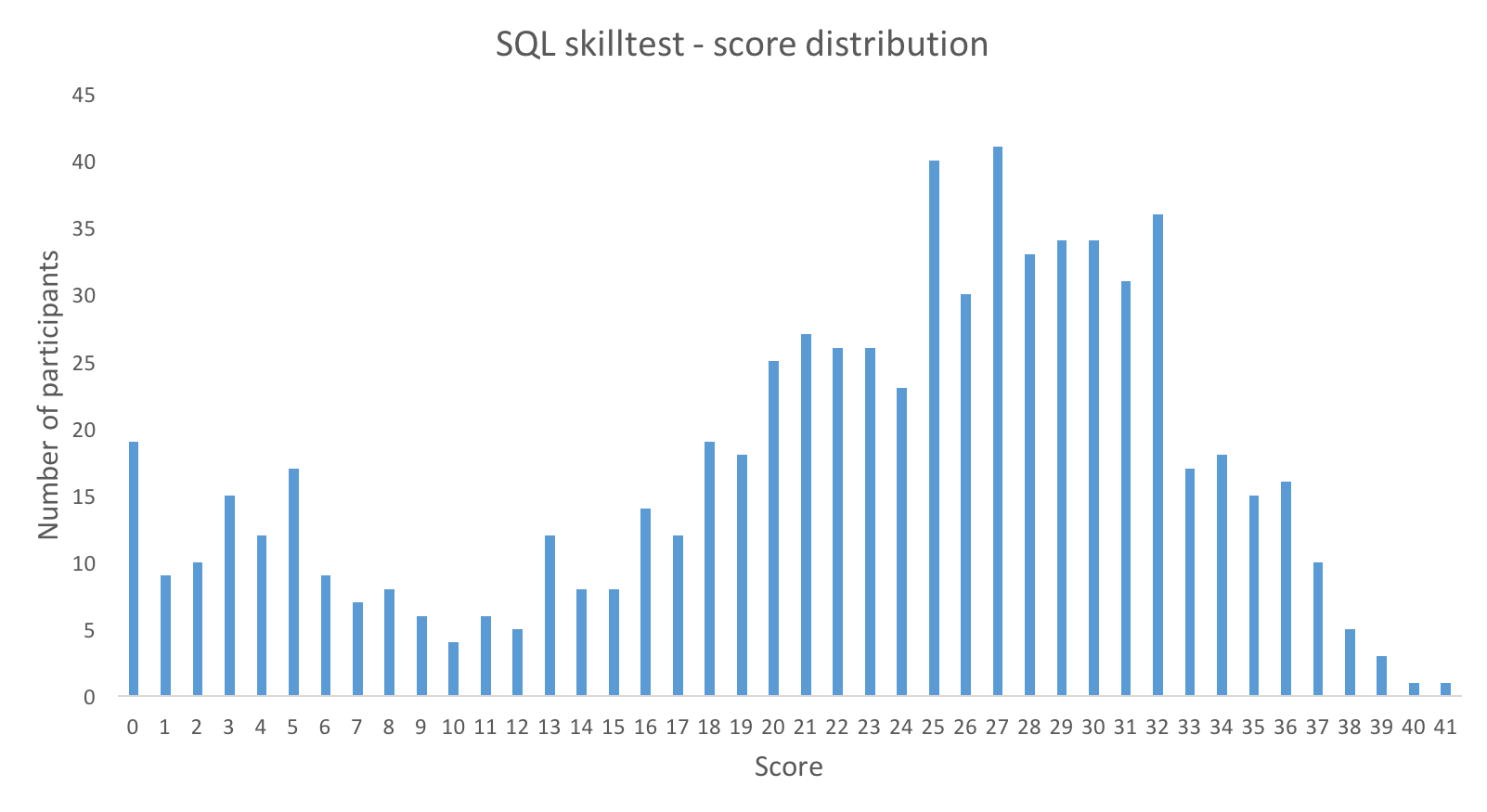You can assess your performance here. More than 700 people participated in the skilltest and the highest score was 41. Here are a few statistics about the distribution.

Overall distribution

Mean Score: 22.32

Median Score: 25

Mode Score: 27

This is an interesting distribution. I think we are seeing 3 different profiles of people here:

• There are ~20 people who did not score at all. They either faced some technical problem or did not like the test or did not know SQL.
• There is another population which looks to have normal distribution between scores 1 to 10. These people either started the competition late and hence could not get enough time or they are just beginners in SQL.
• Third population looks to have distribution between scores 10 and 41 and looks like a representative of people in industry. For this group, mean is 25.8 and standard deviation ~ 6.5 . So any one with a score of more than 32 is in top 16% of the population.

How much did you score and where do you fit?

Basics of SQL and RDBMS – must have skills for data science professionals

SQL commands for Commonly Used Excel Operations

1) Which of the following is the correct order of occurrence in a typical SQL statement?

A. select, group by, where, having

B. select, where, group by, having

C. select, where, having, group byselect, having, where, group by

D. select, having, where, group by

Solution: B

“Where” always comes before “group by” and “having” always comes after “group by”.

Question Context: 2 to 12

STUDENT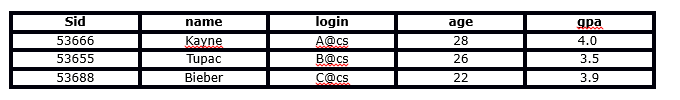ENROLLED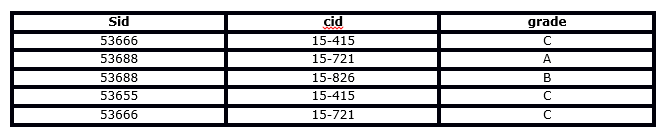Q2) Which of the following is the correct outcome of the SQL query below?

`Query: SELECT cid FROM ENROLLED WHERE grade = 'C'`

A. Extract the course ids(cid) where student receive the grade C in the course

B. Extract the unique course ids(cid) where student receive the grade C in the course

C. Error

D. None of these

Solution:  A

The query will extract the course ids where student receive the grade “C” in the course.

Q3) Which of the following is the correct outcome of the SQL query below?

`Query: SELECT DISTINCT cid FROM ENROLLED WHERE grade = 'C'`

A. Extract the course ids where student receive the grade C in the course

B. Extract the Distinct course ids where student receive the grade of C in the course

C. Error

D. None of these

Solution: B

By using DISTINCT keyword you can extract the Distinct course ids where student receive the grade of C in the course.

Q4) Which of the following is the correct outcome of the SQL query below?

`Query: SELECT name, cid FROM student, enrolled WHERE student.sid = enrolled.sid AND enrolled.grade = 'C'`

A. Returns the name of all students and their corresponding course ids

B. Returns the name of students and their corresponding course id where they have received grade C

C. Error

D. None of these

Solution: B

The above query first joined the ENROLLED and STUDENT tables then it will evaluate the WHERE condition and then it will return the name of students and corresponding course id where they received the grade of C.

Q5) Which of the following is the correct outcome of the SQL query below?

``Query: `SELECT student.name, enrolled.grade FROM student, enrolled WHERE student.sid = enrolled.sid AND enrolled.cid = '15-415' AND enrolled.grade IN ('A', 'B')`

A. Returns the name, grade of the students who took course ’15-415′ and got a grade’ A’ or ‘B’ in that course

B. Returns the name, grade of the students who took the course ’15-415′ but didn’t get grade ‘A’ or ‘B’ in that course

C. Error

D. None of these

Solution: A

The above query first joined the ENROLLED and STUDENT tables then it will evaluate the where condition and then it will return the name, grade of the students, those took 15-415 and got a grade ‘A’ or ‘B’ in the course.  But for the given two tables it will give zero records in output.

Q6) Which of the following query will find all the unique students who have taken more than one course?

`A. SELECT DISTINCT e1.sid FROM enrolled AS e1, enrolled AS e2 WHERE e1.sid != e2.sid AND e1.cid != e2.cid`

`B. SELECT DISTINCT e1.sid FROM enrolled AS e1, enrolled AS e2 WHERE e1.sid = e2.sid AND e1.cid = e2.cid`

`C. SELECT DISTINCT e1.sid FROM enrolled AS e1, enrolled AS e2 WHERE e1.sid != e2.sid AND e1.cid != e2.cid`

`D. SELECT DISTINCT e1.sid FROM enrolled AS e1, enrolled AS e2 WHERE e1.sid = e2.sid AND e1.cid != e2.cid`

Solution: D

Option D would be a right option. This query will first apply self join on enrolled table and then it evaluate the condition e1.sid = e2.sid AND e1.cid != e2.cid.

Q7) Which of the following statement will add a column ‘F_name’ to the STUDENT table?

`A. ALTER TABLE Student add column ( F_name varchar(20));`

`B. ALTER TABLE Student add F_name varchar(20);`

`C. ALTER TABLE Student add (F_name varchar(20));`

`D. ALTER TABLE Student add column (F_name);`

Solution: B

ALTER TABLE command allows a user to add a new column to a table. Option B is correct syntax of ALTER to add a column in the table.

Q8) Which of the following query(s) will result in a successful insertion of a record in the STUDENT table?

`Query1: INSERT INTO student (sid, name, login, age, gpa) VALUES (53888, ‘Drake’, ‘[email protected]’, 29, 3.5)`

`Query2: INSERT INTO student VALUES (53888, ‘Drake’, ‘[email protected]’, 29, 3.5)`

A. Both queries will insert the record successfully

B. Query 1 will insert the record successfully and Query 2 will not

C. Query 2 will insert the record successfully and Query 1 will not

D. Both queries will not be able to insert the record successfully

Solution: A

Both queries will successfully insert a row in table student. The Query 1 is useful when you want to Provide target table, columns, and values for new tuples and Query 2 is a Short-hand version of insert command

Q9) ‘Sid’ in “ENROLLED” table is ‘Foreign Key’ referenced by ‘Sid’ in “STUDENT” table. Now you want to insert a record into the ENROLLED table.

Which of the following option(s) will insert a row in ENROLLED table successfully?

1. `INSERT INTO ENROLLED values(53667, '15-420', 'C');`
2. `INSERT INTO ENROLLED values(53666, '15-421', 'C');`
3. `INSERT INTO ENROLLED values(53667, '15-415', 'C');`
4. `INSERT INTO ENROLLED values(53666, '15-415', 'C');`

A. 1 and 3

B. Only 3

C. 2 and 4

D. Only 4

Solution: C

Option 2 and 4 will run successfully because in ENROLLED table’s ‘Sid’ column you can insert those values which are present in STUDENT’s table ‘Sid’ columns due to foreign key.

Q10) Consider the following queries:

`Query1: select name from enrolled LEFT OUTER JOIN student on student.sid = enrolled.sid;`
` Query2: select name from student LEFT OUTER JOIN enrolled on student.sid = enrolled.sid;`

Which of the following option is correct?

A. Queries 1 and 2 will give the same results

B. Queries 1 and 2 will give different results

C. Query 1 will produce an error and Query 2 will run successfully

D. Query 2 will produce an error and Query 1 will run successfully

Solution: A

In (LEFT, RIGHT or FULL) OUTER joins, order matters. But both query will give the same results because both are dependent on records present in table and which column in selected.

Q11) Which of the following statements will modify the data type of “Sid” column in ENROLLED table?

Note: There is no foreign key relationship between tables STUDENT and ENROLLED.

`A. ALTER TABLE ENROLLED MODIFY (sid varchar(100));`

`B. ALTER TABLE ENROLLED MODIFY sid varchar(100);`

`C. ALTER TABLE ENROLLED MODIFY column (sid varchar(100));`

`D. ALTER TABLE ENROLLED MODIFY attribute (sid varchar(100));`

Solution: A

The “ALTER TABLE MODIFY” is used to modify column definition in a table. So option A is correct.

Q12) Which of the following statement will remove the ‘Sid’ column from the ENROLLED table?

Note: There is no foreign key relationship between tables STUDENT and ENROLLED.

`A. ALTER TABLE ENROLLED DROP (sid varchar(10) );`

`B. ALTER TABLE ENROLLED DROP COLUMN (sid varchar(10) );`

`C. ALTER TABLE ENROLLED DROP COLUMN Sid;`

`D. ALTER TABLE ENROLLED MODIFY (sid);`

Solution: C

The “ALTER TABLE DROP COLUMN” can be used to drop a column from the table. So Option C is the right answer.

Q13) Which of the following command(s) is /  are related to transaction control in SQL?

A. ROLLBACK

B. COMMIT

C. SAVEPOINT

D. All of the above

Solution: D

All are related to transaction control in SQL.

Q14) Which of the following is true for a primary key?

A. It can take a value more than once

B. It can take null values

C. It can’t take null values

D. None of these

Solution: C

In a relational schema, there exist only one primary key and it can’t take null values. So option C is the correct answer.

Q15) What is the difference between a primary key and a unique key?

A. Primary key cannot be a date variable whereas unique key can be

B. You can have only one primary key whereas you can have multiple unique keys

C. Primary key can take null values but unique key cannot null values

D. None of these

Solution: B

You can create a date variable as a primary key in table. In relational schema, you can have only one primary key and there may be multiple unique key present in table. Unique key can take null values.

Q16) Which of the following statement(s) is/are true for UPDATE in SQL?

1. You can update only a single table using UPDATE command
2. You can update multiple tables using UPDATE command
3. In UPDATE command, you must list what columns to update with their new values (separated by commas).
4. To update multiple targeted records, you need to specify UPDATE command using the WHERE clause.

Select the correct option:

A. 1, 3 and 4

B. 2, 3 and 4

C. 3 and 4

D. 1 only

Solution: A

Options are self-explanatory.

Q17) Which of the following is true for TRUNCATE in SQL?

A. It is usually slower than DELETE command

B. It is usually faster than DELETE command

C. There is no comparison between DELETE & TRUNCATE

D. Truncate command can be rolled back

E. None of these

Solution: B

TRUNCATE is faster than delete bcoz truncate is a ddl command so it does not produce any rollback information and the storage space is released while the delete command is a dml command and it produces rollback information too and space is not deallocated using delete command.

Q18) Which of the following statement is correct about ‘CREATE TABLE’ command while creating a table?

A. We need to assign a datatype to each column

B. We have flexibility in SQL. We can assign a datatype to column even after creating a table

C. It is mandatory to insert atleast a single row while creating a table

D. None of these

Solution: A

Each column must possess behavioral attributes like data types and precision in order to build the structure of the table.

Q19) Which of the following are the synonyms for ‘column’ and ‘row’ of a table?

1. Row = [Tuple, Record]
2. Column = [Field, Attribute]
3. Row = [Tuple, Attribute]
4. Columns = [Field, Record]

Select the correct option:

A. 1 and 2

B. 3 and 4

C. Only 1

D. Only 2

Solution: A

In DBMS records are also known as tuple and rows. And columns are known as attributes and fields.

Q20) Which of the following operator is used for comparing ‘NULL’ values in SQL?

A. Equal

B. IS

C. IN

D. None of Above

Solution: B

In SQL if you want to compare a null value you need to use IS statement.

Q21) Which of the following statement(s) is/are true about “HAVING” and “WHERE” clause in SQL?

1. WHERE” is always used before “GROUP BY” and HAVING after “GROUP BY”
2. WHERE” is always used after “GROUP BY” and “HAVING” before “GROUP BY”
3. “WHERE” is used to filter rows but “HAVING” is used to filter groups
4. “WHERE” is used to filter groups but “HAVING” is used to filter rows

Select the correct option:

A. 1 and 3

B. 1 and 4

C. 2 and 3

D. 2 and 4

Solution: A

HAVING is performed after GROUP BY. If you have to apply some conditions to get results. you need to use WHERE before group by.

Q22) Identify, which of the following column “A” or “C” given in the below table is a “Primary Key” or “Foreign Key”?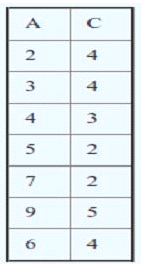Note: We  have defined ‘Foreign Key’ and ‘Primary Key’ in a single table

A. Column ‘A’ is Foreign Key and Column ‘C’ is Primary Key’

B. Column ‘C’ is Foreign Key and Column ‘A’ is ‘Primary Key’

C. Both can be ‘Primary Key’

D. Based on the above table, we cannot tell which column is ‘Primary Key’ and which is ‘Foreign Key’

Solution: B

Column A is taking unique values and column A doesn’t have null values. So it can be considered as Primary key of this table. Whereas B is the example of foreign key because all values present in this column are already present in column A.

Q23) What are the tuples additionally deleted to preserve reference integrity when the rows (2,4) are deleted from the below table. Suppose you are using ‘ON DELETE CASCADE’.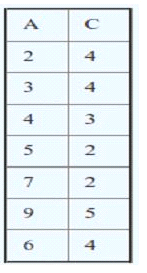Note: We have defined ‘Foreign Key’ and ‘Primary Key’ in single table

A. (5,2) , (7,2), (9,5)

B. (5,2) , (7,2)

C. (5,2) , (7,2), (9,5), (3,4)

D. (5,2) , (7,2), (9,5),(6,4)

Solution: A

When (2,4) is deleted. Since C is a foreign key referring A with delete on cascade, all entries with value 2 in C must be deleted. So (5, 2) and (7, 2) are deleted. As a result of this 5 and 7 are deleted from A which causes (9, 5) to be deleted.

Q24) Suppose you are given a table/relation “EMPLOYEE” which has two columns (‘Name’ and ‘Salary’). The Salary column in this table has some NULL values. Now, I want to find out the records which have null values.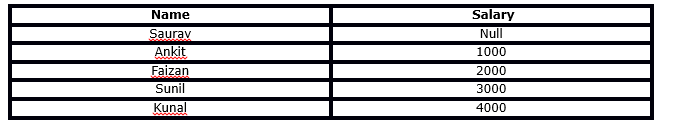What will be the output for the following queries?

`Query 1. SELECT * FROM EMPLOYEE WHERE Salary <> NULL;`

`Query 2. SELECT * FROM EMPLOYEE WHERE Salary = NULL;`

A. Query 1 will give last 4 rows as output (excluding null value)

B. Query 2 will give first row as output (only record containing null value)

C. Query 1 and Query 2 both will give the same result

D. Can’t say

Solution: C

If we compare(<>, =) Salary it will give 0 records. Below are the following reasons:

1. Salary = Null is unknown
2. Salary <> Null is unknown

Q25) What is the difference between TRUNCATE, DELETE and DROP? Which of the following statement(s) is/ are correct?

1. DELETE operation can be rolled back but TRUNCATE and DROP operations cannot be rolled back.
2. DELETE operation cannot be rolled back but TRUNCATE and DROP operations can be rolled back.
3. DELETE is an example of DML (Data Manipulation Language) but remaining are the examples of DDL (Data Definition Language).
4. All are an example of DDL

Select the correct option:

A. 1 and 3

B. 2 and 3

C. 1 and 4

D. 2 and 4

E. None of the above

Solution: A

Options are self-explanatory.

Q26) Tables A, B have three columns (namely: ‘id’, ‘age’, ‘name’) each. These tables have no ‘null’ values and there are 100 records in each of the table.

Here are two queries based on these two tables ‘A’ and ‘B’:

`Query1: SELECT A.id FROM A WHERE A.age > ALL (SELECT B.age FROM B WHERE B.name = 'Ankit')`

`Query2: SELECT A.id FROM A WHERE A.age > ANY (SELECT B.age FROM B WHERE B.name = 'Ankit')`

Now, which of the following statement is correct for the output of each query?

A. The number of tuples in the output of Query 1 will be more than or equal to the output of Query 2

B. The number of tuples in the output of Query 1 will be equal to the output of Query 2

C. The number of tuples in the output Query 1 will be less than or equal to the output of Query 2

D. Can’t say

Solution: C

ANY and ALL operate on subqueries that return multiple values. ANY returns true if any of the subquery values meet the condition. But in case of ALL it will returns the records if all conditions are true. So options C is correct.

Q27) What is true about relation (table) in different normal forms (1NF, 2NF, 3NF)?

1. If a relation satisfies the conditions of 1NF. It will automatically satisfy the conditions of 2NF
2. If a relation satisfies the conditions of 2NF. It will automatically satisfy the conditions of 1NF
3. If a relation satisfies the conditions of 3NF. It will automatically satisfy the conditions of 1NF
4. If a relation satisfies the conditions of 2NF. It will automatically satisfy the conditions of 3NF

Select the correct option:

A. 1 and 2

B. 2 and 3

C. 1 and 3

D. 2 and 4

Solution: B

If a relation is satisfying higher normal forms, it automatically satisfies lower normal forms also. For example, if a relation is satisfying kNF it will automatically satisfy gNF where g<=k.

28) Suppose you want to compare three keys (‘Primary Key’, ‘Super Key’ and ‘Candidate Key’) in a database. Which of the following option(s) is/are correct?

1. Minimal super key is a candidate key
2. Only one Candidate Key can be Primary Key
3. All super keys can be a candidate key
4. We cannot find “Primary Key” from “Candidate Key”

Select the correct option:

A. 1 and 2

B. 2 and 3

C. 1 and 3

D. 2 and 4

E. 1, 2 and 3

Solution: A

Options are self-explanatory

Q29) Consider a relation R with the schema R (A, B, C, D, E, F) with a set of functional dependencies F as follows:

Which of the following will be the output of DA+?

Note: For any X, X+ is closure of X.

A) DA

B) DAE

C) ABCD

D) ABCDEF

Solution: B

(DA)+ =  DAE

30) Suppose you have a table “Loan_Records”.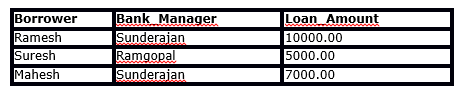`SELECT Count(*)  FROM  ( (SELECT Borrower, Bank_Manager FROM   Loan_Records) AS S NATURAL JOIN (SELECT Bank_Manager, Loan_Amount FROM   Loan_Records) AS T );`

What is the output of the following SQL query?

A. 4

B. 5

C. 8

D. 10

Solution: B

Temporary table S is given below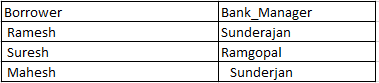Temporary table T is given below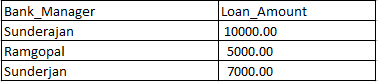If you apply natural join on both tables (S and T) and evaluate the condition on ‘Bank_Manager’. You will get the following intermediate table after apply

natural join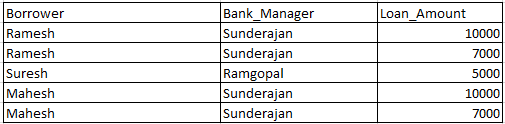“Sunderjan” appears two times in Bank_Manager column, so their will be four entries with Bank_Manager as “Sunderjan”. So count(*) will give the 5 output in outer query.

Q31) Is “SELECT” operation in SQL equivalent to “PROJECT” operation in relational algebra?

A. Yes, both are equivalent in all the cases

B. No, both are not equivalent

Solution: B

In relational algebra ‘PROJECT’ operation gives the unique record but in case of ‘SELECT’ operation in SQL you need to use distinct keyword for getting unique records.

Table: AV1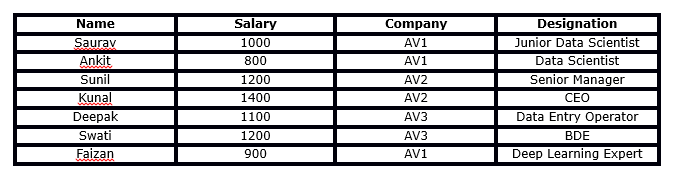Questions  32-36 are based on the above table.
Q32) What will be the output of following query?

`Query 1: Select name from AV1 where name like '%a%'`

1. Saurav, Ankit, Kunal, Deepak, Swati, Faizan
2. Saurav, Kunal , Deepak ,Swati , Faizan
3. Kunal, Deepak ,Swati , Faizan
4. None of above

Ans: B

Solution: The query will search for records in column ‘Name’ which will have atleast one ‘a’ and ‘Like’ operation is case sensitive so A will not be the answer. Hence B is true.

Table: AV1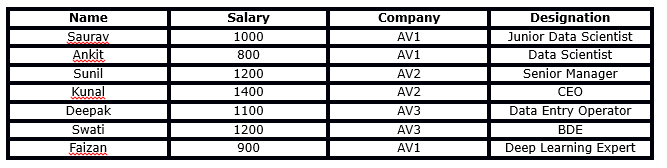Q33) What will be the output for the below query?

`Query: SELECT Name from AV1 where Name LIKE '%______%';`

Note: The above operation contains 6 underscores (‘_’) used with LIKE operator.

A. It will return names where number of characters in names are greater than or equals to 6

B. It will return names where number of characters in names are greater than 6

C. It will return names where number of characters in names are less than or equals to 6

D. It will give an error

Solution: A

The query will search for records in column ‘Name’ where the number of characters in names are greater than or equal to 6.

Q34) What will be the output of the below query?

`Query: SELECT Company, AVG(Salary) FROM AV1 HAVING AVG(Salary) > 1200 GROUP BY Company WHERE Salary > 1000 ;`

A.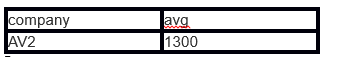B.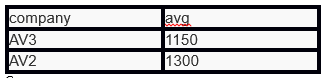C.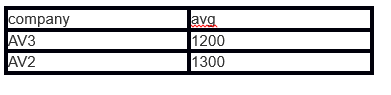D. None of these

Solution: D

This query will give the error because ‘WHERE’ is always evaluated before ‘GROUP BY’ and ‘Having’ is always evaluated after ‘GROUP BY’

Q35) What will be the output for the below Query 1 and Query 2?

`Query 1: SELECT MAX(Salary) FROM AV1 WHERE Salary < (SELECT MAX(Salary) from AV1);`
`Query 2: WITH S AS (SELECT Salary, ROW_NUMBER() OVER(ORDER BY Salary DESC) AS RowNum FROM AV1) SELECT Salary FROM S WHERE RowNum = 2;`

A. Query 1 output = 1200 and Query 2 output =1200

B. Query 1 output = 1200 and Query 2 output =1400

C. Query 1 output = 1400 and Query 2 output =1200

D. Query 1 output = 1400 and Query 2 output =1400

Solution: A

Both queries will generate the second-highest salary in AV1 which is 1200. Hence A is right option.

Q36) Consider the following relational schema.

`Students(rollno: integer, sname: string)`

`Courses (courseno: integer, cname: string)`

`Registration (rollno: integer, courseno: integer, percent: real)`

Now, which of the following query would be able to find the unique names of all students having score more than 90% in the courseno 107?

A. SELECT DISTINCT S.sname FROM Students as S, Registration as R WHERE R.rollno=S.rollno AND R.courseno=107 AND R.percent >90

B. SELECT UNIQUE S.sname FROM Students as S, Registration as R WHERE R.rollno=S.rollno AND R.courseno=107 AND R.percent >90

C. SELECT sname FROM Students as S, Registration as R WHERE R.rollno=S.rollno AND R.courseno=107 AND R.percent >90

D. None of these

Solution: A

Option A is true, Option B will give the error (“UNIQUE” is not used in SQL) and in option C unique names will not be the output.

Q37) Consider the relation T1 (A, B) in which (A, B) is the primary key and the relation T2 (A, C) where A is the primary key. Assume there are no null values and no foreign keys or integrity constraints.

Now, which of the following option is correct related to following queries?

`Query 1: select A from T1 where A in (select A from T2)`

`Query 2: select A from T2 where A in (select A from T1)`

A. Both queries will definitely give the same result

B. Both queries may give the same result

C. Both queries will definitely give a different result

D. None of these

Solution: B

For the same values ( values should be unique) for the column A in tables T1 and T2. Query 1 and Query 2 will give the same output. Hence B is true

Q38) Which of the following option is correct about following queries?

`Query 1. SELECT emp.id, department.id FROM emp NATURAL JOIN department`

`Query 2. SELECT emp.id, department.id FROM department NATURAL JOIN emp`

A. Both queries will give same outputs

B. Both queries will give different output

C. Need table structure

D. None of these

Solution: A

For Natural joins, the order doesn’t matter. The queries will return same results.

Q39) Indexing is useful in a database for fast searching. Generally, B-tree is used for indexing in a database. Now, you want to use Binary Search Tree instead of B-tree.

Suppose there are numbers between 1 and 100 and you want to search the number 35 using Binary Search Tree algorithm. Which of the following sequences CANNOT be the sequence for the numbers examined?

A. 10, 75, 64, 43, 60, 57, 55

B. 90, 12, 68, 34, 62, 45, 55

C. 9, 85, 47, 68, 43, 57, 55

D. 79, 14, 72, 56, 16, 53, 55

Solution: C

In BST on right side of parent number should be greater than it, but in C after 47, 43 appears that is wrong.

Q40) If an index scan is replaced by sequential scan in SQL, then what will happen?

Note: Number of observations is equal to 1 million.

A. Execution will be faster

B. Execution will be slower

C. Execution will not be affected

D. None of these

Solution: B

The addition of the index made the query execution faster since the sequential scan is replaced by the index scan.

Q41) Suppose you have a csv file which has 3 columns (‘User_ID’, ‘Gender’, ‘Product_ID’) and 7,150,884 rows. You have created a table “train” from this file in SQL.

Now, you run Query 1 as given below and get the following output:

`Query 1: EXPLAIN select * from train where Product_ID = 'P00370853';`

OUTPUT:

`QUERY PLAN`
`--------------------------------------------------------------`
`Seq Scan on train (cost=0.00..79723.88 rows=16428 width=68)`
`Filter: ((product_id)::text = 'P00370853'::text)`
`(2 rows)`

You have now created Product_ID column as an index in train table using the below SQL query:

CREATE INDEX product_ID ON train(Product_ID)

And, you run Query 2 (same as Query 1) on “train” and get the following output.

`Query 2: EXPLAIN select * from train where Product_ID = 'P00370853';`

OUTPUT:

`QUERY PLAN`
`-------------------------------------------------------------------------------`
`Bitmap Heap Scan on train (cost=829.53..40738.85 rows=35754 width=68)`
`Recheck Cond: ((product_id)::text = 'P00370853'::text)`
`-> Bitmap Index Scan on product_id (cost=0.00..820.59 rows=35754 width=0)`
`Index Cond: ((product_id)::text = 'P00370853'::text)`
`(4 rows)`

Which query will take less time to execute?

A. Query 1

B. Query 2

C. Both queries will take the same time

D. Can’t say

Solution: B

For Query Plan for Query 1 execution time is 79723.88 and for Query Plan of Query 2 execution time is  40738.85. So Query 2 is taking less time.

Q42)  Suppose you have a CSV file which has 3 columns (‘User_ID’, ‘Gender’, ‘product_ID’) and 7150884 rows. You have created a table “train” from this file in SQL.

Now, you run Query 1 (mentioned below):

`Query1: EXPLAIN SELECT * from train WHERE product_ID like '%7085%';`

Then, you created product_ID columns as an index in ‘train’ table using below SQL query:

`CREATE INDEX product_ID ON train(Product_ID)`

Suppose, you run Query 2 (same as Query 1) on train table.

`Query 2: EXPLAIN SELECT * from train WHERE product_ID like '%7085%';`

Let T1 and T2 be time taken by Query 1 and Query 2 respectively. Which query will take less time to execute?

A. T1>T2

B. T2>T1

C. T1~T2

D. Can’t say

Solution: C

The addition of the index didn’t change the query execution plan since the index doesn’t help for the ‘LIKE’ query.

Q43) Suppose you have a table ‘Employee’. In Employee table, you have a column named Salary. Now, you applied Query1 on Employee table.

`Query 1: SELECT * FROM Employee where Salary*100 > 5000;`

After that, you created an index on Salary columns and then you re-run the Query 2 (same as Query 1).

`Query 2:  SELECT * FROM Employee where Salary*100 > 5000;`

Here, Query 1 is taking T1 time and Query 2 is taking T2 time.

Which of the following is true for the queries time?

A. T1 > T2

B. T2 > T1

C. T1 ~ T2

D. Can’t say

Solution: C

The addition of the index didn’t change the query execution plan.  The index on rating will not work for the query (Salary * 100 > 5000). Theoretically it might work in this case, but obviously the system is not “smart” enough to work that way; But you can create an index on (Salary * 100) which will help.

Q44) Suppose you are given a table ‘words’. The table has 2 columns ‘id’ and ‘word’.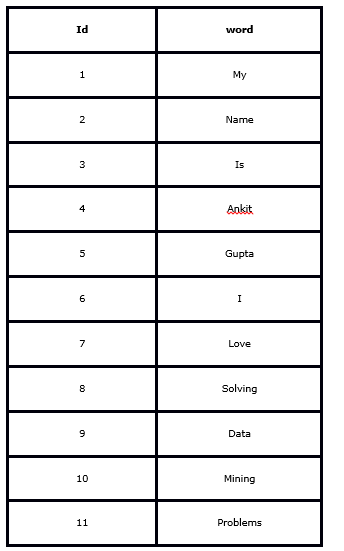What will be the output for the below query?

Query:  select c1, c2, c3 from ( select id,  lag(word) over (order by id) as c1, word as c2, lead(word) over (order by id) as c3 from words ) as t where c2 = ‘Mining’ or c2 = ‘Problems’;

A.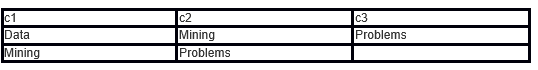B. Error

C.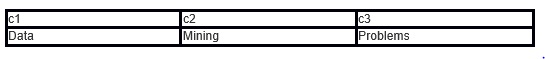D. None of these

Solution: A

Q45) What is true for ‘View’ in SQL?

1. It can help in providing security
2. It can be used for hiding complexity
3. If there are more than one table involved in the view, we cannot perform (Data Manipulation Language) DML queries
4. When you drop the base table. View becomes inactive.

Select the correct option:

A. 1 and 3

B. 2 and 4

C. 1, 3 and 4

D. All of these

Solution: D

All options are correct.

Q46) Suppose I created a table called ‘avian’ using below SQL query:

Query :` CREATE TABLE avian ( emp_id SERIAL PRIMARY KEY, name varchar);`

Now, I want to insert some records in the table avian:

`Query1: INSERT INTO avian (name) VALUES(`‘FRAZY`');`

`Query2: INSERT INTO avian (name) VALUES(`‘ANKIT`');`

`Query3: INSERT INTO avian (name) VALUES('SUNIL');`

`Query4: INSERT INTO avian (name) VALUES('SAURAV');`

Which of the following will be the output of the below query?

`Query: Select * FROM avian;`

A.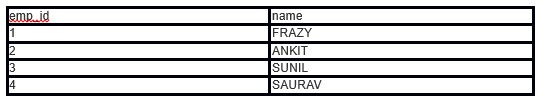B.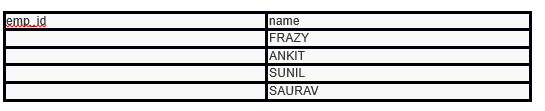C. Error

D. None of these

Solution: A

At the time of table creation Avian, we have used SERIAL for ’emp_id’ which autoincrement emp_id whenever you insert a record in table avian. Hence A is true.

### End Notes

I hope you enjoyed taking the test and you found the solutions helpful. The test focused on conceptual knowledge of SQL.

We tried to clear all your doubts through this article but if we have missed out on something then let me know in comments below. If you have any suggestions or improvements you think we should make in the next skilltest, let us know by dropping your feedback in the comments section.

### Learn, compete, hack and get hired!

You can also read this article on our Mobile APP•RK says:

Hi
in Q11: You have given Ans: A
1) option A and D are same
2) Answer should be option B?

•RK says:

Ignore the above comment

•Benny says:

Hi Ankit,
Thanks for the sols.
Q29 : didnt understand the qn. could you explain the qn and sol breifly.
Q38 : Query2 will throw an error since emp is not an alias for employee. Solution ans is wrong
Q39: For BST, i believe the series should be ordered. Can you breifly explain the sol again.

Regards,
B

•Ankit Gupta says:

Hi Benny,

Thanks for noticing it.

For question number 29 refer the links:
2. http://www.edugrabs.com/closure-of-a-set-x/

In question number 39, query is updated as per the skill test.
If you consider the nodes after 47 in option C, we take the right sub-tree for 68. The right sub-tree can contain only those nodes which are larger than 47. But 43 is less than 47 ( because 68 is inserted at the right side of 47 and then 43 will be inserted at left side of 68, 43 suppose to be left side of 47 but it is right side of 47)

•Philip says:

Can you increase the time of test at least for 4 hours . I really wanted to attempt the quiz but hardly I got 10 mins to solve , because I logged in late. They are many more things coming up. As this test are on weekend if you can increase the hour span then it will be good. Thanks

•Ankit Gupta says:

Hi Philip,

Feedback taken.

Best!
Ankit Gupta

•cxchan2 says:

Hi Ankit,
Good job on the sql skill test. It was more like a sql refresher. I am not sure why there was no option of going back to correct or review a question. If we would have had that option, it would have been more helpful.

•Ankit Gupta says:

Hi Philip,

Feedback taken.

Best!
Ankit Gupta

•Jack Ma says:

This question is not related to directly related to this question.

Is it possible to add the print button to the page?
A lot of articles are great. But when I try to print it out. The format is terrible and print friendly.

•Ankit Gupta says:

Hi Jack,

There are many extensions available for browsers which will do this job.

Best!
Ankit Gupta

•Jack Ma says:

Thank you.
I do find one extension called print friendly&pdf. It is much better.

•Hunaidkhan Pathan says:

Hi Jack,
You can use Google chrome browser and right click on any of the page you want and click on print -> it does have good options to change the print view. Also google chrome allows to save the page as PDF .

•Jack Ma says:

Hi Hunaidkhan,

I am using chrome. But the original print is not that good for some article, there has some overlap. But i use the extension now. it helps me a lot.

•Pushpita says:

Very Informative. Loved the questions and really good explanation…

•Ankit Gupta says:

Hi Pushpita,

Best!
Ankit

•Ankit Sabinkar says:

This was my first Hackathon and I really enjoy it.

Lot more to come in future.

AV Team is doing an amazing job. Thank you !!!

•Ankit Gupta says:

Hey Ankit,

Regards,
Ankit

•Mario says:

Can I re-use these questions/answers to form a bank of question/answers for quizzes in an academic course?

•Ankit Gupta says:

Hi Mario,

•Mohamed Hindam says: